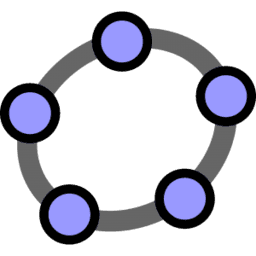# Learn Calculator Suite

Welcome to the GeoGebra Calculator Suite! Learn how to use this full powered math app including solving equations, derivatives and integrals with CAS, function graphing, tables, statistics, dynamic geometric 2D and 3D constructions and more:
• Plot functions, equations, curves and surfaces in 2D and 3D
• Build tables of values
• Solve equations
• Find derivatives and integrals
• Explore transformations with sliders
• Get special points of functions: roots, min, max, intersections
• Analyze different types of regressions
• Create geometric constructions and 3D objects
• Measure lengths, perimeters and areas
• Create and analyze traces of points and loci
• Experience sliders, points, graphs and geometry all working together
• Investigate construction steps to get a deeper understanding
• Calculate probability distributions und conduct statistical tests
• Switch between Graphing, Geometry, CAS, 3D Calculator and Probability